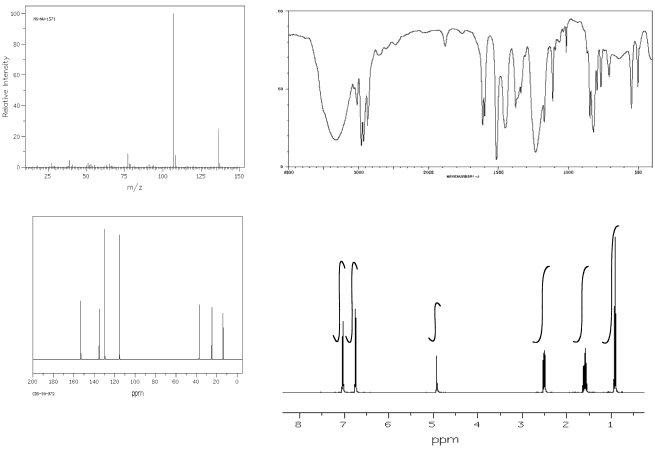# Determine Structure with Combined Spectra

There are a many ways we can use NMR spectroscopy to analyse compounds. One common application is in determination of an unknown structure. Given the MS, IR, 13C and 1H NMR spectra, what might be the structure of an unknown sample?

• identify at least three peaks in the IR spectrum. Which peaks seem to tell you the most information about this compound?
• if you are working on a formal proof of structure, on a class test or a lab report, you may be required to enter your data in a table correlating wavenumber with peak assignment:
 cm-1 asst

For example, a student might obtain the following IR spectrum.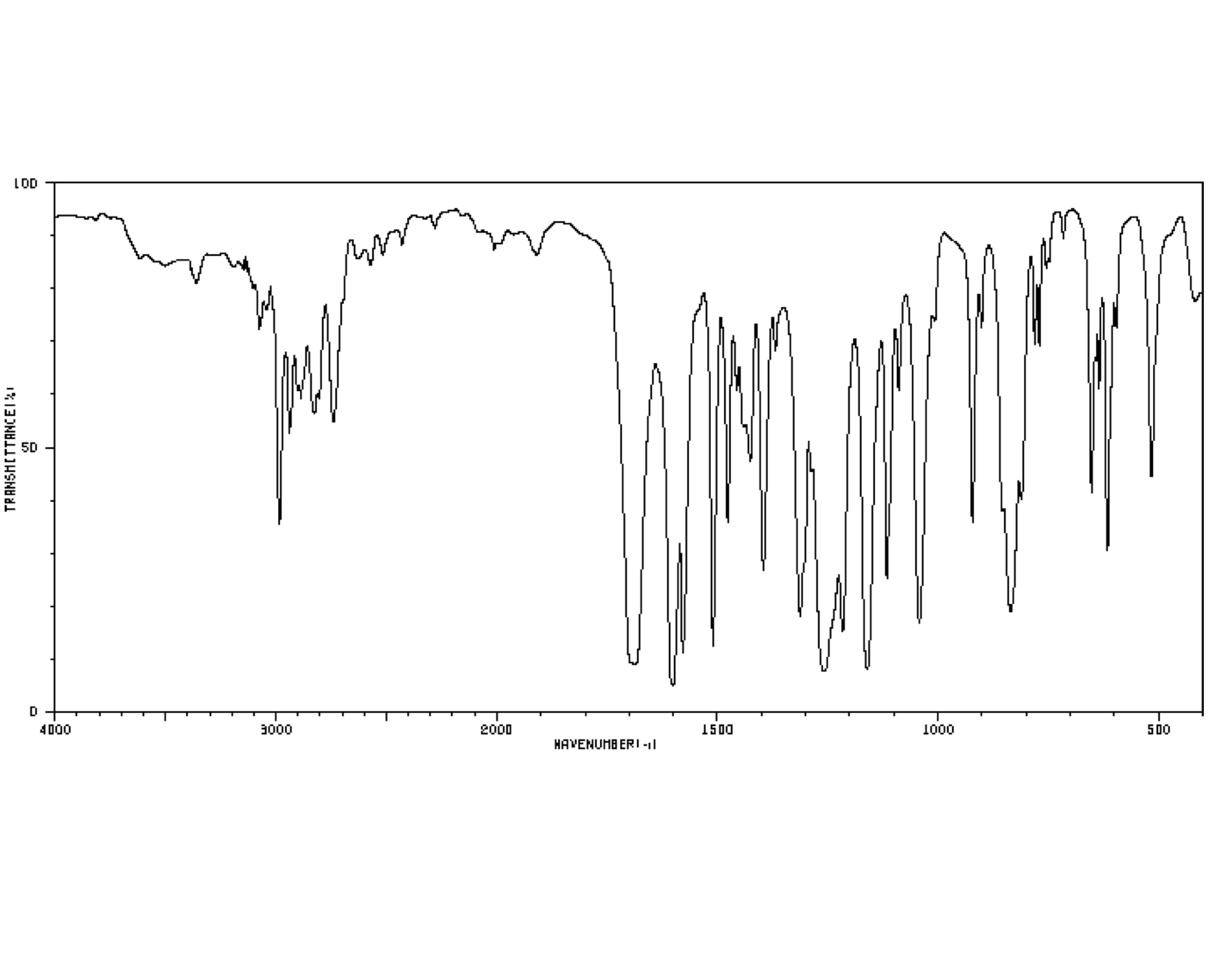From that information, she constructs the following table. She might even write this table, by hand, directly on her spectrum. She makes useful notes on the edges, and might even include some guesses, which she later crosses out, but does not erase. She is assisted in this task by consulting an IR table, that suggests what some of these peaks might mean.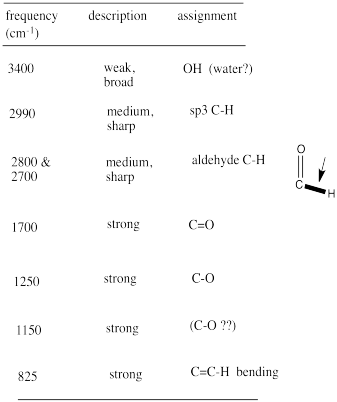Remember:

• make special note of what atoms are present in the compound: C, H, N, O...
• also note your initial ideas about specific functional groups that may be present.
• if you are unsure of an assignment, put a question mark beside it to signal this uncertainty.
• some data may need to be discarded later if it is not consistent with other data.

Look at the 13C spectrum.

• How many different carbons are there, based on the number of peaks in the spectrum? This is the first step in estimating the molecular formula.
• Do you have reason to believe there is symmetry in the structure? In the entire compound or just part of it? Adjust the number of carbons you think you are dealing with.
• As in IR spectroscopy, begin assigning peaks, either on the spectrum or, if required, in a table:
 ppm asst

For example, a student might obtain the following 13C NMR spectrum: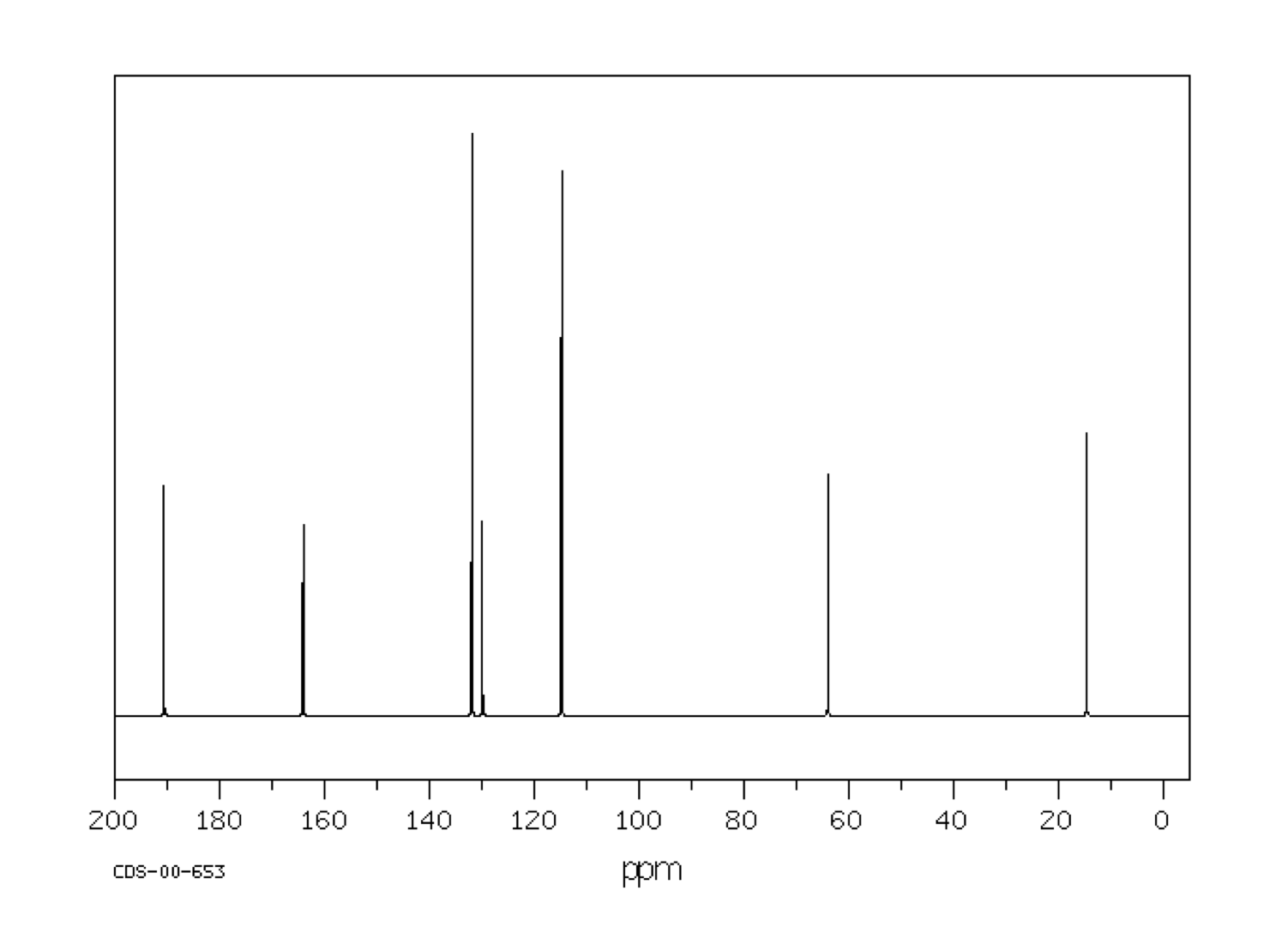From that information, she puts together the following table: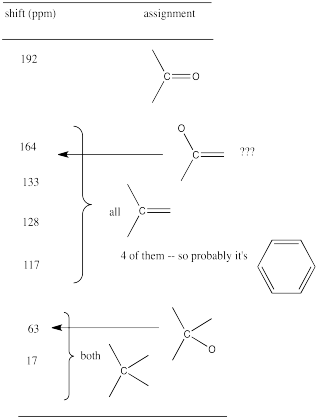Remember:

• you will be able to assign all peaks in the NMR spectrum, not just a few like in IR.

You can get more information on the formula from the 1H NMR spectrum and the mass spectrum.

• In the 1H NMR spectrum, what does the sum of the integrations suggest about the number of hydrogens?
• At this point you may have a formula, CxHyOz. What would be the mass of a compound with this formula?
• Compare this mass to the mass spectrum. Does it match?
• If not, consider whether a common atom (such as oxygen) is missing from your formula, or if there might be symmetry that you have missed.
• Does the mass spectrum suggest the presence of a nitrogen (odd molecular weight) or chlorine or bromine (isotope pattern)?

Once you have the molecular formula, the number of possible structures is automatically limited. The number of units of unsaturation can help you narrow down the possibilities.

• The ratio of C : H in a saturated, acyclic hydrocarbon is n : 2n+2.
• Each pair of H missing from the formula corresponds to a multiple bond or a ring.
• The presence of oxygen (which is divalent) does not alter the C : H ratio.
• The presence of halogen (which is monovalent) means there is one H missing.
• The presence of a nitrogen (which is trivalent) means there is an extra H in the formula.
• In other words, C4H8O has one ring or double bond just like C4H8, and so do C4H7Br and C4H9N.

As in 13C NMR, you should be able to assign all peaks in the 1H NMR spectrum. You may be able to do so by making notes on the spectrum. If you think you know the structure, you may be able to draw it and note which peak belongs with which proton.

A formal proof of structure might require a table of assignments.

 ppm int mult partial structure assignment

• This table demonstrates your ability to read the spectrum. Can you decide what ratio of protons is suggested by the integral line? Can you decide whether a peak is a quartet?
• The partial structure column should explain the shift, integration and multiplicity for the peak in that row. It should not show any other information from elsewhere in the structure. This restriction forces you to demonstrate a thorough understanding of the data in a way that "getting the right answer" does not.
• The partial structure column is best filled in with drawings, not words. The drawing is a partial structure.
• Because the partial structure will show the protons absorbing at the shift in that row as well the neighbouring protons, you need to distinguish between them in your picture. Most people circle or underline or make bold the protons that show up at the shift given in that row.
• When finished with the partial structure column, you should be able to link the partial structures together to make an entire structure in the assignment column.

An example of a spectrum and its accompanying data table is given below. Here is the spectrum: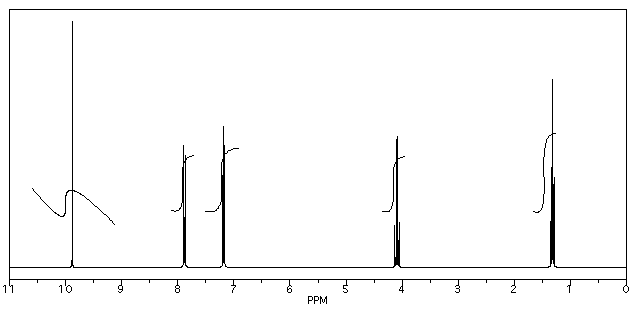Here is a data table: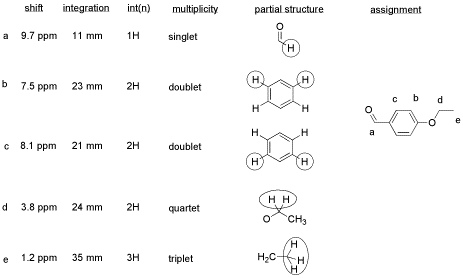Things to note:

• This student has used two integration columns instead of just one.
• The first column shows the integral measured from the spectrum. She probably used a ruler.
• The second column, which she called int(n), contains a convenient ratio taken from the raw data. This ratio is easier to use in her assignments.
• Also note that the peak at 9.7 ppm does not have a very good integral. There is either a "phasing" or a "level & tilt" problem here that can be corrected using the NMR software, but this is sometimes difficult to do. If she had taken an automatic printout of this integral measurement, she would have gotten a strange number; in this case, it would be about -5, because the end of the integral line is lower than the start. It clearly isn't a negative number of hydrogens, though. She has instead measured the vertical rise in the integral and recorded that; it isn't perfect, but is a fair estimate in this case.

### Problem NMR.11.

Using the approach outlined above, build a case for the structure of the compound represented by the data below.